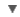POS
WORDNET DICTIONARY
CIDE DICTIONARY
OXFORD DICTIONARY
Link, Gadget and Sharewatt

RELATED WORDS :

:
Noun

CIDE DICTIONARY

wattn. [From the distinguished mechanician and scientist, James Watt.].
A unit of power or activity equal to 107 C.G.S. units of power, or to work done at the rate of one joule a second. An English horse power is approximately equal to 746 watts.  [1913 Webster]

OXFORD DICTIONARY

watt, n. the SI unit of power, equivalent to one joule per second, corresponding to the rate of energy in an electric circuit where the potential difference is one volt and the current one ampere.

Idiom
watt-hour the energy used when one watt is applied for one hour.
Usage
Symb.: W.
Etymology
J. Watt, Sc. engineer d. 1819
copyright © 2012 Yayasan Lembaga SABDA (YLSA) | To report a problem/suggestion